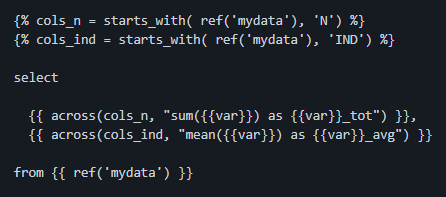dbtplyrdbt example code

dbt_dplyr is an dbt add-on package bringing dplyr semantics to SQL. In particular, it mimics dplyr's across() function and the select helpers to easily apply operations to a set of columns programmatically determined by their names.

dplyr (>= 1.0.0) has helpful semantics for selecting and applying transformations to variables based on their names. For example, if one wishes to take the sum of all variables with name prefixes of N and the mean of all variables with name prefixes of IND in the dataset mydata, they may write:

summarize(
mydata,
across( starts_with('N'), sum),
across( starts_with('IND', mean)
)

This package enables us to similarly write dbt data models with commands like:

{% cols_n = dbtplyr.starts_with( ref('mydata'), 'N') %}
{% cols_ind = dbtplyr.starts_with( ref('mydata'), 'IND') %}

select

{{ dbtplyr.across(cols_n, "sum({{var}}) as {{var}}_tot") }},
{{ dbtplyr.across(cols_ind, "mean({{var}}) as {{var}}_avg") }}

from {{ ref('mydata') }}

which dbt then compiles to standard SQL.